• Scripting and Programming for OpenFOAM

How many of these names have you heard of? Click on the names to know more about them!New boundary conditions, utility and solver programming in OpenFOAM

### OpenFOAM: Programming

#### Disclaimer:

This content is not approved or endorsed by OpenCFD Limited, the producer of the OpenFOAM software and owner of the OpenFOAM and OpenCFD trademarks.
One of the strengths of OpenFOAM is that new solvers and utilities can be created by its users with some prior knowledge of the underlying method, physics and programming techniques involved. This tutorial by Tommaso Lucchini from Department of Energy Politecnico di Milano serves to generate this understanding. OpenFOAM is a C++ library used primarily to create executable, known as applications. The applications are further categorized into two groups: 'solvers' which are each designed to solve a specific problem in continuum mechanics and 'utilities' to perform tasks that involve data manipulation. Wikki Ltd is provider and major contributor to foam-extend, the community version of the OpenFOAM software. A solver needs to be compiled into a binary file whereas boundary conditions need to be compiled into a shared obeject library. The shared object library can be dynamically linked to any solver without re-compiling.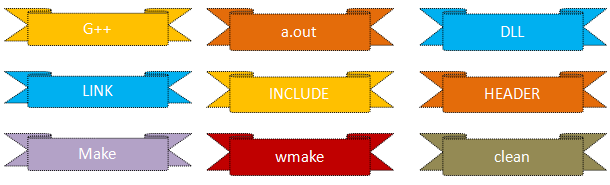#### OpenFOAM code for the N-S equation:

Note that the specifications fvm and fvc are selected by the user from the fvSchemes dictionary in the system dictionary. Here fvm::laplacian means an implicit finite volume discretization for the Laplacian operator, and similarly for fvm::div for the divergence operator. On the other hand, fvc::grad means an explicit finite volume discretization for the gradient operator. fvm (Finite Volume Method) returns a matrix, fvc (Finite Volume Calculus) refers the field variables. The parentheses ( - ) means a product of the enclosed quantities, including tensor products. Similarly, & denotes dot product of two vectors in OpenFOAM. The code to solve Navier-Stokes equation described below is written for demonstration of fvm and fvc class. fvm returns a fvMatrix and fvc evaluate derivative based on known GeometricField values and returns appropriate geometricField.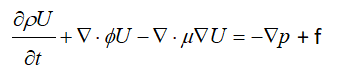Use of &: phi = (fvc::interpolate(U) & mesh.Sf()) calculates flux through faces of a control volume by calculating dot product of vectors U.A

OpenFOAM like any other numerical solver, converts the Partial Differential Equations (PDEs) into a set of linear algebraic equations, [A] {x} = {b}, where {x} and {b} are volFields (geometricField). [A] is a fvMatrix which is created by the discretization of a geometricField and inherits the algebra of its corresponding field. It supports many of the standard algebraic matrix operations.

```Solve (
fvm::ddt(rho, U) + fvm::div(phi, U) - fvm::laplacian(mu, U) == f - fvc::grad(p)
);
```
solve() performs inversion to solve [A]{x} = {b} for one step. 'U' is a volVectorField defined on a mesh – a discretised representation of the field variable 'u'. fvm::ddt, fvc::grad construct entries in matrix equation of form [A] {x} = {b}. solve() performs inversion to solve for one step {x} = [A]-1 {b}.
• fvc::ddt(A) = ∂A/∂t
• fvc::ddt(rho, A) = ∂(ρA)/∂t
• fvc::d2dt2(rho, A) = ∂(ρ.∂A/∂t)/∂t
• fvc::laplacian(s, A) = ∇(s.∇A)
• fvc: explicit evaluation of derivatives (i.e. based on known values). fvm: implicit evaluation of derivativesTo solve the transient diffusion equation: ∂φ/∂t = κ∇2φ, an Euler implicit implementation of this would read as follows where fvm class to discretise the Laplacian term implicitly.:

solve(fvm::ddt(phi) == kappa*fvm::laplacian(phi))

An explicit implementation would read where the fvc class to discretise the Laplacian term explicitly.

solve(fvm::ddt(phi) == kappa*fvc::laplacian(phi))

The Crank Nicholson scheme can be implemented by the average of implicit and explicit terms:

solve (fvm::ddt(phi) == kappa*0.5*(fvm::laplacian(phi) + fvc::laplacian(phi)))

Similarly, the statement to solve k-equation in OpenFOAM is given by
```solve (
fvm::ddt(k) + fvm::div(phi, k) - fvm::laplacian(nu() + nut, k) ==
); ```
Here k-equation is expressed as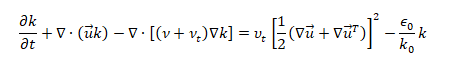Su:Implicit evaluation of the source term in the equation. SuSp(phi, A): Implicit/Explicit source term in equation, depending on sign of phi.

#### Pressure-based Equation

Mass conservation:

```solve (
fvm::ddt(rho)
+ fvc::div(phi)
); ```

Momentum equation:

```fvVectorMatrix UEqn  (
fvm::ddt(rho, U)
+ fvm::div(phi, U)
- fvm::laplacian(mu, U)
);

Energy equation:

```solve (
fvm::ddt(rho, e)
+ fvm::div(phi, e)
- fvm::laplacian(mu, e)
==
- p*fvc::div(phi/fvc::interpolate(rho))
);
T = e/Cv; ```

#### Density-based compressible flow solver

```solve (
fvm::ddt(rho)
+ fvm::div(phiv, rho)
);

solve (
fvm::ddt(rhoU)
+ fvm::div(phiv, rhoU)
==
);

solve (
fvm::ddt(rhoE)
+ fvm::div(phiv, rhoE)
==
- fvc::div(phiv2, p)
); ```

OpenFOAM can be used to solve equations governed by theory of elasticity (solid mechanics) as well.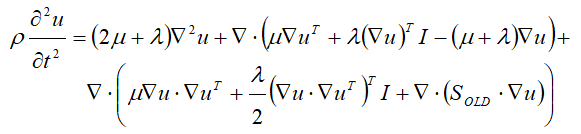This equation can be solved in OpenFOAM by:
```fvVectorMatrix UEqn (
rho * fvm::d2dt2(U) == fvm::laplacian(2*mu + lambda, U)
+ fvc::div (
)
);	```
```fvScalarMatrix TEqn (
fvm::ddt(T) + fvm::div(phi,T) - fvm::laplacian(DT,T)  // = 0
);
Teqn.solve(); ```

Can you write a solver which supercedes all those defined separately for single phase incompressible flows?

####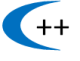Syntax and Conventions

A short and detailed presentation on C++ programming methods and its implementation in OpenFOAM can be found here.

C++ is a strongly type language: checks for types of all data and functions and gives compiler errors if they do not fit. C++ is a statically typed [that error checking is done only during compilation], compiled [a separate application called compiler is needed and executable needs to be created], case-sensitive, free-form [unlike FORTRAN or Python where indentation specifies a block of code it does not recognize end of the line as a terminator . Thus, it does not matter where a statement in a line is put] programming language that supports procedural, object-oriented programming. An interpreted language such as Python may also be object-oriented but error checking is done during run-time and no compilation is required. C++ has backward compatibility to C, that is the programs written in C can be compiled in C++ environment without any modification. The Java Virtual Machine and Python interpretor are written in C++

github.com/ UnnamedMoose/ BasicOpenFOAMProgrammingTutorials provides a good starting point for those looking to develop apps in OpenFOAM. Some codes from this repository has also been included in later section of this page.

Ubuntu on WSL (Windows Sub-system for Linux) uses g++ to compile C++ codes. Use command `whereis g++` to check if g++ is installed or not. If not, use command `sudo apt-get install g++` to install the compiler. g++ hello.cpp: to complie the code, use command ./a.out to run the compiled code (a.out is the default name of executable). g++ hello.cpp -o hello: to name the executable 'hello'. Use ./hello to run the executable created.

C++ is a family of programming language designated as "Object Oriented". Object orientation focuses on the 'objects' instead of the functions. An 'object' belongs to a class of objects with the same features or attributes or properties. For example, 'student' can be a 'class' of objects and roll number, student name, grade ... would be the attributes. The class of objects defines:

• The construction of the object
• The destruction of the object
• Attributes of the object (member data)
• Methods which manipulate the object (member functions)
Object members are either functions or data. 'public' attributes are visible from outside the class. 'private' attributes (default) are visible only within the class.
```class foamEqn {
public:  //declaration of public members
int m;
private: //declaration of hidden members
}```
std::cout << "Hello" << " C++" = Hello C++ - the << is a concatenation operator. The outputs from following programs would be 'mat.txt' and 'bc.txt'.
```#include <iostream>
void fileNames(string fname) {
cout << fname << "*.txt\n";
}

int main() {
fileNames("mat");
fileNames("bc");
return 0;
} ```
The :: operator - It is called the scope-resolution operator and by prefixing a type-name with this, it tells compiler to look in the global namespace for the type. In other words, :: links either of a variable, function, class, typedef... to a namespace or to a class. If there is no left hand side before :: then it refers to the global namespace. Thus, in 'fvm::laplacian', fvm is a namespace and laplacian is a function (it can also be another namespace of a variable or an onject). Foam: namespace for OpenFOAM, constant: namespace for collection of constants, fv: namespace for finite-volume, fvc (Finite Volume Calculus): namespace of functions to calculate explicit derivatives - given a field creats a new field, fvm (Finite Volume Method): namespace of functions to calculate implicit derivatives returning a matrix.
• Statements end with a semi-colon, blocks of code such as those in loops are enclosed in curly braces { ... }
• There are essentially three uses of the preprocessor in C++: directives, constants, and macros.
• Directives are commands that tell the preprocessor to skip part of a file, include another file or define a constant or macro. Directives always begin with a hash sign (#) and for readability they are placed flush to the left of the sentence.
• All other uses of the preprocessor involve processing (#)defined constants or macros. Typically, constants and macros are written in ALL CAPITAL LETTERS to indicate they are special.
• Conditional Compilation: options that tell preprocessor to remove lines of code before compiler take over the files. They include #if, #elif, #else, #ifdef, and #ifndef. An #if or #if/#elif/#else block or a #ifdef or #ifndef block must be terminated with a closing #endif.
• #include: this directive tells the preprocessor to grab the text of a file and place it directly into the current file.
• #ifndef: this directive stands for IF Not DEFined
• #define: define a constant. e.g. #define PI (3.14156)
• OpenFOAM screen output is very similar to rudimentary C++ with its std::cout, std::nl and std::endl replaced by Foam::Info, Foam::nl, and Foam::endl
• << and >> are output and input operators and 'endl' is a manipulator that generates a new line (equivalent to \n). Instead of using 'cin' and 'cout', OpenFOAM defined a new output stream 'Info' and it is recommended to use this one instead since it takes care of write-outs for parallel simulations.
• namespace is a method to distinguish two variables or functions having same names. It is analogous to roll numbers to differentiate two students having exactly same name.
• << can also be used to combine text and variable though '+' operator is used to concatenate two strings.
• OpenFOAM has implemented (inherited) few variables to suit the programming requirement.
• label = integer (int in C++): the size of variable types in bytes are not explicitly defined in C++
• scalar = float or double
• word = single word (inherited rom C++ string object)
• string = quoted text
• list = array bounded by () e.g. (1 2 4 8)
• prefixList = array of type 'prefix'e.g. labelList, wordList, scalarList
• using namespace Foam::constant → The 'using' directive here is used to refer to a particular item within a namespace.
• Foam::scalar Foam::noiseFFT::p0 = 1e-5 → Here the variable p0 in scopes 'Foam::scalar' and 'Foam::noiseFFT' are assigned a value of 1e-5.
• typedef: create a new name for an existing type. e.g. typedef int single; → single is another name for int, typedef float real → real is another name for float. Note that the term 'void' represents the absence of type. In case of a 'function' it tells that the function does not return anything.
• Constants refer to fixed values that the program cannot alter, they are also called literals. Constants can be defined either using #define preprocessor or 'const' keyword.
• x = &myObj defines x as pointer to object myObj. & implies object is a reference i.e. it refers to an object of the same class. x->read() accesses the member function read(). e.g. turbulence->correct() in \$FOAM_SOLVERS/ incompressible/ pimpleFoam/ pimpleFoam.C
• -> is used to call a member data (or function) through a pointer. OpenFOAM uses 'pointer' functionality to make run-time choices (such as selection of turbulence model) possible.
• A '*' after an object name implies that a pointer should be constructed.
• 'forAll' defined in UList.H is an alias to 'for-loop' defined to march through all entries of a list of objects of any class.
• If an argument variable should be changed inside a function, the type of the argument must be a reference i.e. void change(double& x1). The reference parameter x1 here will be a reference to the argument to the function instead of a local variable in the function.
 Pointer Reference Holds memory address of another object Alias of another object Can be re-assigned Must be assigned at initialization

Operators: The usual precedence-rules apply to all of the following.

• &: Inner product for vectors and tensors, ^: Cross product of two vectors
• %: Modulo operator
• && ||: The logical and and or operators, - : Unary negation, ! : Logical negation
• < > >= <=: Relational comparisons, == != : : Equality and inequality-operators
• ? and : Ternary operator for cond ? a : b. The condition must evaluate to bool, the fields a and b must be of the same type.
• The keyword bool can be used to force a boolean conversion of scalar values. A threshold of -/+ 0.5 is used to define true/false.
• pos(x): if x > 0: 1.0 else 0.0, pos0(x) : if x ≥ 0: 1.0 else 0.0
• neg(x): if x < 0: 1.0 else 0.0
• neg0(x): if x ≤ 0: 1.0 else 0.0
• sign(x): if x is positive: 1.0 else if x is negative: -1.0
• mag(x): Absolute value |x| Implemented for all data types. Yields a scalar. Can be also be used to convert a bool to a scalar.
• magSqr(x): Square of the magnitude |x|^2 Implemented for all non-logical data types. Yields a scalar
• pow(x,y): Power x^y, exp(x): Exponential function e^x, log(x): Natural logarithm, log10(x): Logarithm base 10, sqr(x): Square x^2, sqrt(x): Square root, cbrt(x) : Cubic root

Concepts in OOP (Object-Oriented Programming)Classes in OpenFOAM:
• 'polyMesh' class holds mesh definition objects whereas polyBoundaryMesh is a list of polyPatches
• To access fields defined at cell centers of the mesh you need to use the class volField which can be accessed by adding the header volFields.H
Example of Scalar Volume Field:
```volScalarField p (   //Create the scalar volume field
IOobject (  //Assign and initialize volField to mesh
"p", runTime.timeName(), mesh,
),
mesh
); ```
Geometric (mesh) information held in fvMesh object. Basic mesh information held includes points, faces, cells and boundaries (eg. points as pointField, cells as cellList) which is read in from constant/polyMesh. fvMesh is also responsible for mesh changes due to mesh motion. Fields of objects (vectors, scalars, tensors) defined at each point on the mesh:
• volScalarField field of scalars (eg. pressure)
• volVectorField field of vectors (eg. velocity)
• volTensorField field of 2nd rank tensors (eg. stress)

####OpenFOAM root folder structure

Use the command [tree -d -L 2 \$FOAM_SRC] to list the folder structure (such as the one shown below) of OpenFOAM src folder. Where '2' specifies level of sub-folders to be listed. Use '1' for only upper level folders.Aliases are defined: \$WM_PROJECT_DIR/etc/config.sh/aliases.
1. src: the core OpenFOAM libraries with *.C and *H files, including include files. Typing 'foam' on the command line will take you to installation folder. Similarly, app = \$WM_PROJECT_DIR/applications = cd \$FOAM_APP and util = \$WM_PROJECT_DIR/applications/utilities. Command alias | grep "FOAM" retrieves following aliases
• alias app='cd \$FOAM_APP'
• alias lib='cd \$FOAM_LIBBIN'
• alias run='cd \$FOAM_RUN'
• alias sol='cd \$FOAM_SOLVERS'
• alias src='cd \$FOAM_SRC'
• alias tut='cd \$FOAM_TUTORIALS'
• alias util='cd \$FOAM_UTILITIES'
2. One can add his/her own aliases such as: alias ubc='cd \$FOAM_SOURCE/finiteVolume/fields/fvPatchFields/derived' - to move to folder containing all boundary conditions.
3. bin: The bin directory contains shell scripts, such as paraFoam,foamNew, foamLog...
4. Other userful aliases can be: alias fv='cd \$FOAM_SRC/finiteVolume', alias usrSrc='cd \$WM_PROJECT_USER_DIR/src', alias fo='cd \$FOAM_SRC/OpenFOAM/db/functionObjects'. Note there should be no whitespace character between 'useSrc' and '=' and make sure that the folder exists.
5. \$FOAM_APP/solvers/: solvers (such as icoFoam, pimpleFoam, sonicFoam ...) and utilities (such as blockMesh, setFields, topoSet ...). Solvers are grouped into 12 categoryies: basic, compressible, DNS, financial, incompressible, multiphase, combustion, discreteMethods, electromagnetics, heatTransfer, lagrangian and stressAnalysis. Move into the folder solver using command 'sol'
6. \$FOAM_SRC/finiteVolume/finiteVolume: this folder contains discretization schemes namely convectionSchemes, ddtSchemes, fv, fvm, d2dt2Schemes, divSchemes, fvc, fvSchemes, fvSolution, gradSchemes, laplacianSchemes, snGradSchemes. Use alias 'fv' to move into \$FOAM_SRC/finiteVolume directory.
7. \$FOAM_SRC/OpenFOAM: This core library includes the definitions of the containers used for the operations, the field definitions, the declaration of the mesh and of all the mesh features such as zones and sets
8. \$FOAM_SRC/TurbulenceModel: contains the source code of turbulence models
9. \$FOAM_APPBIN: The path to the folder containing the executables. Note that the \$FOAM_APP refers to the folder containing the source codes.
10. \$FOAM_TUTORIALS: sample cases (simulation data) to demonstrate OpenFOAM functionalities, tutorial folder by typing 'tut' on command line
11. test: cases that test and validate individual models or aspects of OpenFOAM functionality. Sample codes to help understand the usage of OpenFOAM libraries
12. etc: The etc directory contains environment set-up files, global controlDict and default thermoData. scripts -foamyHexMeshDict, mesh, meshQualityDict, postProcessing, setConstraintTypes, solvers, surface
13. doc: documentation such as user guides in PDF format, Doxygen
14. In addition to these folders, the root folder also contains a file call 'Allwmake' and a directory called 'wmake'. These are 'make' utility for compilations.
15. \$WM_PROJECT_DIR: Full path to locate binary executables of OpenFOAM release
16. Use command <find -iname "sampleDict"> to find a file named sampleDict. To search a folder named 'pimpleFoam' in folder '\$WM_PROJECT_DIR' use command: find \$WM_PROJECT_DIR –type d -name "*pimpleFoam*" where * is known as wildcard character. To find a file replace 'd' with 'f'. Similarly, command "find \$FOAM_SRC -iname \*fixedValue\*.[CH]" lists all the occurrences of fixedValue.C and fixedValue.H files in folder \$FOAM_SRC.
17. \$WM_PROJECT_DIR/src/OpenFOAM/lnInclude: list of *.H and *.c files linked to specific utility.
18. \$WM_PROJECT_USER_DIR is the user's directory for running OpenFOAM cases.
19. All the source codes related to boundary conditions can be found in the src files of respective boundary condition in finiteVolume/ fields/ fvPatchField/ derived under \$FOAM_SRC. In this folder, you can also check which all boundary conditions types are available with the installed version of OpenFOAM.
20. Environment variables pointing to local (user's) workspace applications and libraries: \$FOAM_PROJECT_USER_DIR, \$FOAM_USER_APPBIN and \$FOAM_USER_LIBBIN
You can check the value of all shortcuts (alias in the world of Linux) and environment variables using 'echo' command. E.g. echo \$FOAM_RUN, echo \$WM_PROJECT_DIR ... A list of OpenFOAM specific environment variable settings used by wmake is as follows:
 \$WM_PROJECT_INST_DIR Installation folder e.g. /opt for OpenFOAM installed in Windows Subsystem for Linux (WSL) \$WM_PROJECT Name of the project being compiled = OpenFOAM \$WM_PROJECT_VERSION Version of the project being compiled: 6 \$WM_PROJECT_DIR Path to executables of OpenFOAM release e.g. /opt /openfoam7 \$WM_PROJECT_USER_DIR Path to executables created by user e.g. /home /amkum78 /OpenFOAM /amkum78-7 \$WM_THIRD_PARTY_DIR Full path to the ThirdParty software directory e.g. /opt /ThirdParty-7 \$WM_ARCH Machine architecture: linux, linux64, linuxIa64, linuxARM7, linuxPPC64, linuxPPC64le \$WM_ARCH_OPTION 32-bit or 64-bit architecture \$WM_COMPILER Compiler being use - e.g. Gcc \$WM_COMPILE_OPTION Compilation option: Debug - debugging, Opt optimisation. \$WM_COMPILER_TYPE Choice of compiler: system, ThirdParty - compiled in ThirdParty directory \$WM_DIR Full path of the wmake directory \$WM_LABEL_SIZE 32 or 64 bit size for labels (integers) \$WM_LABEL_OPTION Int32 or Int64 compilation of labels \$WM_LINK_LANGUAGE Compiler used to link libraries and executables C++. \$WM_MPLIB Parallel communications library: SYSTEMOPENMPI - system version of openMPI \$WM_OPTIONS e.g. linux64GccDPInt32Opt, linuxGccDPInt64Opt

The 'platforms' directory contains the binaries of the applications and libraries after their compilation. They are organized according to the Linux environment used during compilation, more specifically the \$WM_OPTIONS environment variable. For example, if \$WM_OPTIONS has the value linux64GccDPInt32Opt it means that the binaries were compiled for Linux, 64-bit, by the gcc compiler, for double precision floats and 32 bit integers. That is set in \$WM_PROJECT_DIR/ etc/config.sh/ settings:

export WM_OPTIONS=\$WM_ARCH\$WM_COMPILER\$WM_PRECISION_OPTION\$WM_LABEL_OPTION\$WM_COMPILE_OPTION

Some of those are set in \$WM_PROJECT_DIR/etc/bashrc:
• export WM_COMPILER=Gcc
• export WM_PRECISION_OPTION=DP
• export WM_COMPILE_OPTION=Opt
while two of them are set in \$WM_PROJECT_DIR/etc/config.sh/settings:
• export WM_ARCH=\$(uname -s)
• export WM_LABEL_OPTION=Int\$WM_LABEL_SIZE
where the last one is set (again) in \$WM_PROJECT_DIR/etc/bashrc: export WM_LABEL_SIZE=32
#####File Structure OpenFOAM
Source code style in OpenFOAM is very consistent and follows Object Oriented Approach to its core. Few salient features are:
• Codes separation into files: *.C and *H
• Comment and indentation style such 4 characters for indentation
• Common approach to common needs e.g. I/O, construction of objects, stream support, handling function parameters, const and non-const access
• Blank lines, no trailing whitespace, no spaces around brackets
• Porting and multiple platform support handled through environment variables
• All tools, compiler versions and paths can be controlled with environment variables
Search online for "OpenFOAM expression expression syntax guide" to get official version of the programming standards implemented / followed in OpenFOAM. The OpenFOAM API Guide provides description of modules such as solvers, boundary conditions....
• OpenFOAM follows object oriented programming where the types (int, double) can be seen as classes and the variables assigned to a type are objects of that class (int a). Object orientation focuses on the objects instead of the functions. An object belongs to a class of objects with the same attributes. The class defines:
• The construction of the object
• The destruction of the object
• Attributes of the object (member data)
• Methods which manipulate the object (member functions)
• There needs to be a *.C file with the solver name that calls all of the necessary libraries as well as initializing the time, meshes and material properties. This file contains the time loop as well as the calls to the individual solvers.
• The other .H files in the solver directory relate to the initialization and the calls within the time loop.
• The Make directory includes a file titled options which "contains the full directory paths to locate the header files … and library names" while the file titled 'files' lists the entire path and name of the solver once it has been compiled. Make/files: names all the source files (.C), it specifies the boundary condition library name and location of the output file.
• The difference between compilation of solver and boundary condition lies in the fact that solvers are compiled into executables and boundary conditions are compiled into shared object libraries which can be linked to any solver without need to recompile for every solver.
• OpenFOAM usage 'wmake' utility for compilation - a reference copy is available in folder '\$FOAM_SRC'.
• OpenFOAM represents scalars, vectors and matrices as tensor fields. A zero rank tensor is a scalar, a first rank tensor is a vector and a second rank tensor is a (3 × 3) matrix. OpenFOAM contains a C++ class library named primitive (\$FOAM_SRC/OpenFOAM/primitives/) which contains classes for the tensor mathematics.
• If a tensor is defined as "tensor T(1, 2, 3, 4, 5, 6, 7, 8, 9)" then T.yz() ≡ T(2,3) = 6.
OpenFOAM follows few naming convention of variables such as "scalar maxValue_" where each variable name ends with an underscoe '_' The file structure is shown below.Typical content of the 'Make/files' is shown below. The Make directory contains instructions for the 'wmake' compilation command.

XiFoam.C

EXE = \$(FOAM_APPBIN)/XiFoam → instructs what the new executable name will be

LIB = \$(FOAM_USER_LIBBIN)/libAcousticAnalogy → instructs what the new library name will be

The compiler searches for the included header files in the following order, specified with the -I option in 'wmake':

1. \$WM_PROJECT_DIR/src/OpenFOAM/lnInclude directory
2. local lnInclude directory
3. local directory
4. platform dependent paths set in files in the \$WM_PROJECT_DIR/wmake/rules/\$WM_ARCH/ directory. e.g. /usr/X11/include and \$(MPICH_ARCH_PATH)/include
5. other directories specified explicitly in the Make/options file with the -I option
The keyword 'EXE' above indicates that it is an executable, in case of libraries such as boundary conditions - the keyword 'LIB' is used. options: specifies directories to search for include files and libraries to link the solver against. Typical content of the 'options' file is as follows. EXE_INC refers to INCLUDE files for EXEcutable (solver) and EXE_LIBS implies INCLUDE files for LIBraries. Note that the directory names are preceeded by the '-I' flag and that the syntax uses the backslash '\' to continue the EXE_INC across several lines, with no '\' after the final entry.

The Make/options file contains the full directory paths and library names using the syntax: EXE_LIBS = -L<libraryPath> -l<libraryName> The directory paths are preceeded by the -L flag, the library names are preceeded by the -l (small L) flag.

Make/options: specifies directories to search for include files and libraries to link the solver against.

```EXE_INC = \
-I\$(LIB_SRC)/finiteVolume/lnInclude \
-I\$(LIB_SRC)/meshTools/lnInclude \
-I\$(LIB_SRC)/sampling/lnInclude

EXE_LIBS = \
-lfiniteVolume \
-lmeshTools \
-lsampling```
/finiteVolume/lnInclude is the symbolic link to the library 'finiteVolume'. Similarly, \$FOAM_SRC/ finiteVolume/ lnInclude/ fvCFD.H is symbolic link to: \$FOAM_SRC/ finiteVolume/ cfdTools/ general/ include/fvCFD.H. The 'lnInclude' directory contains the "symblic link" to all the files and headers required during compilation. All the 'include' files such as #include "turbulenceModel.H", #include "pimpleControl.H" ... will be seached in this folder. Relevant 'Make' system variables are:
• EXE: Location for the executable. Use \$FOAM_APPBIN or \$FOAM_USER_APPBIN
• LIB: location for the library. Use \$FOAM_LIBBIN or \$FOAM_USER_LIBBIN
• EXE_INC: location of include paths. Use \$LIB_SRC. Each library soft-likts all files into lnInclude directory for easy inclusion of search paths
• EXE_LIBS, LIB_LIBS: Link libraries for executables or other libraries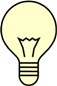Note:

It is important to use Make files as per the versions of OpenFOAM you are using. For example, Make/options in V3.x and V8.0 used in simpleFoam are copied below. The Make/options does not make any reference to TurbulenceModels and has undergone significant changes. The folder and keyword TurbulenceModels has been replaced with MomentumTransportModels.

V3.x / V5.x / V7.x

```EXE_INC = \
-I\$(LIB_SRC)/TurbulenceModels/turbulenceModels/lnInclude \
-I\$(LIB_SRC)/TurbulenceModels/incompressible/lnInclude \
-I\$(LIB_SRC)/transportModels \
-I\$(LIB_SRC)/transportModels/incompressible/singlePhaseTransportModel \
-I\$(LIB_SRC)/fvOptions/lnInclude \
-I\$(LIB_SRC)/finiteVolume/lnInclude \
-I\$(LIB_SRC)/meshTools/lnInclude \
-I\$(LIB_SRC)/sampling/lnInclude

EXE_LIBS = \
-lturbulenceModels \
-lincompressibleTurbulenceModels \
-lincompressibleTransportModels \
-lfiniteVolume -lmeshTools -lfvOptions -lsampling ```

V8.0

```EXE_INC = \
-I\$(LIB_SRC)/MomentumTransportModels/momentumTransportModels/lnInclude \
-I\$(LIB_SRC)/MomentumTransportModels/incompressible/lnInclude \
-I\$(LIB_SRC)/transportModels/lnInclude \
-I\$(LIB_SRC)/finiteVolume/lnInclude \
-I\$(LIB_SRC)/meshTools/lnInclude \
-I\$(LIB_SRC)/sampling/lnInclude

EXE_LIBS = \
-lmomentumTransportModels \
-lincompressibleMomentumTransportModels \
-lincompressibleTransportModels \
-lfiniteVolume -lmeshTools -lfvOptions -lsampling ```

The wmake script is executed by typing: wmake <optional Arguments> <optional Directory>. The <optional Arguments> is the directory path of the application that is being compiled. Typically, wmake is executed from within the directory of the application being compiled, in which case <optional Directory> can be omitted. To build an application executable no <optional Arguments> are required.

Following <optional Arguments> may be specified for building libraries. Argument Type of compilation are:

• lib: Build a statically-linked library
• libso: Build a dynamically-linked library
• libo: Build a statically-linked object file library
• jar: Build a JAVA archive
• exe: Build an application independent of the specified project library

wclean

On execution, wmake builds a dependency list file with a .dep file extension and a list of files in a Make/\$WM_OPTIONS directory. To remove these files the user can run the wclean script. This script located in folder \$WM_DIR cleans up the wmake control directory Make/\$WM_OPTIONS and removes the 'lnInclude' directories generated for libraries.By design, OpenFOAM is intended to be used inside Linux OS and a sound knowledge of this operating system will help one manoeuvre the folders easily. E.g. cp -r is a command required to copy a folder. -r option stands for recursive copy. Similarly, \$(!!) can be used to access the output from last command. 'tree' command list the content of current folder in a hierarchical tree structure: `tree -d -L 1 \$WM_PROJECT_DIR`

#### FVM solution methodBefore further moving into the OpenFOAM program structure, let's look at the governing equations and methods adopted for their numerical solutions.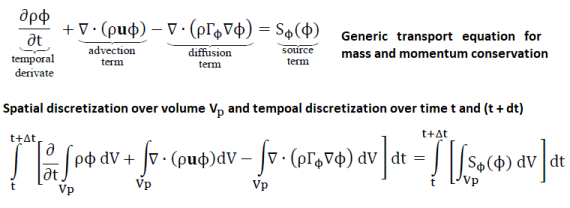The integration over a cell volume need to be converted into surface intergral over the face of the cells using Gauss theorem as explained below.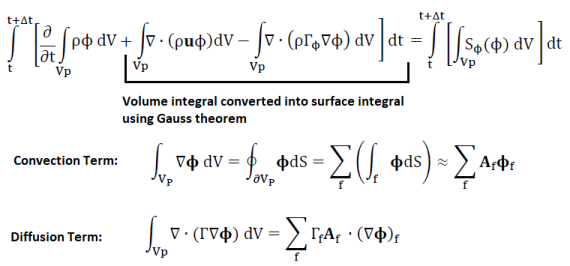∭ρT dx.dy.dz can be implemented as:
``` volVectorField cen = mesh.C();
scalar sumRT = 0.0;
forAll(cen, k) {
sumRT = sumRT + (mesh.V()[k] * rho[k] * T[k]));
} ```
3D version of Burgers equation ∂u/∂t = 1/2 . ∇.(uu) = ν∇2u can be implemented in OpenFOAM as:
```fvVectorMatrix UEqn (
fvm::ddt(U) + 0.5*fvm::div(phi, U) - fvm::laplacian(nu, U)
); UEqn.solve();  ```
UEqn.relax will add relaxation factos to the equation.
Let's look at some of the settings in fvScheme dictionary. Note that the variable φ defined above is a generic representation of u, v, w ... and does not represent 'phi' defined below.
```ddtSchemes   {               //ddtScheme is a 'class' under namespace 'fv'
default         Euler;
}

default         Gauss linear;
}

divSchemes   {
default          none;
div(phi,k)       bounded Gauss upwind;
div(phi,epsilon) bounded Gauss upwind;       //phi = U.A = Flux field
div(phi,R)       bounded Gauss upwind;
div(R)           Gauss linear;
div(phi,nuTilda) bounded Gauss upwind;       //nuTilda = Spalart-Allmaras model
div((nuEff*dev2(T(grad(U))))) Gauss linear;  //nuEff = nuLam + nuTurb
}```
dev() calculates the deviatoric part of the matrix: ADEV = A - AHYD = A - 1/3 × tr(A)I. OpenFOAMr versions like 4.x onwards, the incompressible solvers will use dev2() [introduced in version 3 for better stability and convergence] otherwise used for compressible flows. The difference between dev2 and dev is the factor two at some position of the code. dev2() calculates the deviatoric part of the matrix: ADEV = A - 2AHYD = A - 2/3 × tr(A)I.
```laplacianSchemes {
default         Gauss linear corrected;
}

interpolationSchemes {
default         linear;
}```
Solvers operate on the lduMatrix class located in folder FOAM_SRC/OpenFOAM/matrices/lduMatrix/ - lduMatrix is a matrix class in which the coefficients of matrix [A] in linear equation [A]{x}={b} are stored as three arrays. One for the lower triangle (l), one for the the diagonal of the matrix (d) and the third array for uppper triangle (u) - hence known as lduMatrix. Inside of lduMatrix.H and lduMatrix.C, the member functions - const scalarField & lower () const, const scalarField & diag () const and const scalarField & upper () const are defined which returns the list of cofficients for the lower, diagonal and upper part of the matrix.

fvSolution: pimpleFoam This dictionary defines the selection of linear solver, smoothing algorithm and convergence tolerance for inner iterative process.

1. BICCG - Diagonal Incomplete LU preconditioned BiCG solver
2. diagonalSolver - diagonal solver for both symmetric and asymmetric problems
3. GAMG - generally known as Geometric-agglomerated Algebraic MultiGrid solver (named Generalised geometric-Algebraic MultiGrid in the user manual)
4. ICC - Incomplete Cholesky preconditioned Conjugate Gradients solver
5. PBiCG - Preconditioned bi-conjugate gradient solver for asymmetric lduMatrices using a run-time selectable preconditioner
6. PCG - Preconditioned conjugate gradient solver for symmetric lduMatrices using a run-time selectable preconditiioner
7. smoothSolver - Iterative solver using smoother for symmetric and asymmetric matrices which uses a run-time selected smoother
Preconditioner and smoother: Preconditioners improves the convergence of the origina system [A]{x}={b} using a matrix [M] and resulting in a new system [M]-1[A]{x} = [M]-1{b}. Smoother on the other hand is to reduce the dependency of number of iterataions on mesh type.
```solvers {
p {
solver           GAMG;
tolerance        1e-7;
relTol           0.01;
smoother         DICGaussSeidel;
}

pFinal {
\$p;
relTol          0;
}

"(U|k|epsilon)"  {
solver          smoothSolver;
smoother        symGaussSeidel;
tolerance       1e-05;
relTol          0.1;
}

"(U|k|epsilon)Final" {
\$U;
relTol          0;
}
}

PIMPLE {
nNonOrthogonalCorrectors 0;
nOuterCorrectors    100; //Outer Loops (Pressure - Momentum Correction )
nCorrectors         2;   //Inner Loops (Pressure Correction )
} ```

volField<Type>: Field defined at cell centres, surfaceField<Type>: Field defined at cell faces, pointField<Type>: Field defined at cell vertices.
 Description Access function Data type Total number of cells mesh.nCells() scalar Total number of internal faces mesh.nInternalFaces() scalar Cell volumes mesh.V() volScalarField Face area normal vectors mesh.Sf() surfaceVectorField Face area magnitudes mesh.magSf() surfaceVectorField Cell center mesh.C() volVectorField Face center mesh.Cf() surfaceVectorField Nodal mesh.points() pointField Classes volScalarField Cell volumes V() surfaceVectorField Face area vector Sf() surfaceScalarField Face area magnitude magSf() volVectorField Cell centres C() surfaceVectorField Face centres Cf() surfaceScalarField Face fluxes Phi() Return central coefficients of an fvVectorMatrix A() H operation source of an fvVectorMatrix H() Return face flux field from an fvScalarMatrix flux() Correct boundary fields of a volVectorField correctBoundaryConditions()

To access fields defined at cell centers of the mesh you need to use the class volField. Information about the mesh: Functions that provide information about the mesh and are used without any arguments.

 Function Domain(s) Description pos() P, V Native positions (P: face centres, V: cell centres) pts() P, V Point positions (P: face points, V: mesh points) fpos() V The face centres area() P, V The face area magnitudes face() P, V The face areaNormal vectors vol() V The cell volumes weightAverage(..) P, V Area or volume weighted average (global) weightSum(..) P, V Area or volume weighted sum (global)Utility statements

• runTime.write(): write to stadard output
• Centre of faces: Cf[faceI].x() / patchName.Cf()[i], Cf[faceI].y() / patchName.Cf()[i], Cf[faceI].z() / patchName.Cf()[i]. E.g. volVectorField centres = mesh.C() creates a vector field names 'centres' which holds X, Y and Z coordinates of the centroids of the mesh cells.
• Access time: this->db().time().value()
• Loop over all faces of a patch: forAll(Cf, faceI) { field[faceI] = ... } Note that forAll is a OpenFOAM macro and can be replaced with standard for loop in C++ with new iterator instead of iterator 'patchI' or 'faceI'. forAll(Cf, faceI) = for (int faceI=0; Cf.size()<faceI; faceI++)
• forAll(U, i) is equivalent to "for (int i=0; patch.size()<i; i++)". zpatch.Cf()[i] - indices ,  and  are used to access the X-, Y- and Z-coordinates respectively.
• volVectorField cen = mesh.C() - create a variable of class 'volVectorField' named 'cen' which holds the centroids of all cells in the mesh.
• surfaceVectorField srf = mesh.Sf() - create a variable of class 'surfaceVectorField' named 'srf' which holds the surface area vector of all faces in the mesh.
• surfaceScalarField smg = mesh.magSf() - create a variable of class 'surfaceScalarField' named 'smg' which holds the magnitude of surface areas of all faces in the mesh.
• surfaceVectorField scn = mesh.Cf() - create a variable of class 'surfaceVectorField' named 'scn' which holds the centroids of all faces in the mesh.
• surfaceScalarField ffx = Phi() - create a variable of class 'surfaceScalarField' named 'ffx' which holds the flux through all the faces the mesh. In other words, phi = mesh.Sf() & Uf where & denotes the dot product.
For loop:
```forAll(Cf, faceI) {
if((Cf[faceI].z() > Z0) && (Cf[faceI].z() < Z1) &&
(Cf[faceI].y() > Y0) && (Cf[faceI].y() < Y1)  ) {
if ( t < 10.0) {
field[faceI] = 1.0;
}
else {
field[faceI] = 5.0;
}
}
}```
Note the following two examples of codes used to write name and size of patches (number of faces) are equivalent:

Code with forAll loop

```forAll(mesh.boundaryMesh(), patchI)
Info << "Patch " << patchI << ": " << mesh.boundary()[patchI].name() << " with "
<< mesh.boundary()[patchI].Cf().size() << " faces. Starts at total face "
<< mesh.boundary()[patchI].start() << endl; ```

Code with standard C++ loop

```for (int i = 0; i < mesh.boundaryMesh().size(); i++)
Info << "Patch " << i << ": " << mesh.boundary()[i].name() << " with "
<< mesh.boundary()[i].Cf().size() << " faces. Starts at total face "
<< mesh.boundary()[i].start() << endl; ```

#include "mathematicalConstants.H" - this file contains definition of constants like pi, e, R ... and can be accessed as mathematicalConstant::pi. The scope-resolution operator such as Foam::pow(X, n) is used to calculate Xn.

createFields.H: declares all the field variables and initializes the solution. Contains field and material property definition used by the equation.

Create a field by reading it from a file:
```volScalarField T (
IOobject (
"T", runTime.timeName(), mesh,
IOobject::AUTO_WRITE
), mesh
); ```

In OOP like C++ used in OpenFOAM, a dot operator is used to access methods and attributes of an object. For example, piple.loop() refers to loop() method defined in object 'pimple'. Similarly, runTime.x() defined below where 'x' stands for attributes of object runTime.

Retrieving data from a dictionary: Communication to disk (files, dictionaries, fields...) is controlled by IOobject. IOdictionary object to interface with 'transportProperties' file, then look-up 'DT' in the dictionary. runTime.constant( ): values read from 'constant' folder, runtime.system() will read IOdictionary from 'system' dictionary / folder, runTime.timeName(): values read from time directories. Provides a list of saved times: runTime.times(). IOobject defines the I/O attributes of entities managed by the object registry.
• When the object is destroyed or asked to write: AUTO_WRITE, NO_WRITE
• runTime is a variable of OpenFOAM class Time: used to control the time-stepping through the code
```IOdictionary transportProperties (
IOobject (
"transportProperties",
runTime.constant(), mesh,
IOobject::NO_WRITE
)
);
dimensionedScalar DT( transportProperties.lookup("DT") ); ```

In the following example NO_READ implies data does not need to be read from any dictionary: rather – construct using ::mag() function specified inside the code. Then write it out to the terminal windows.

```volumeScalarField magU (
IOobject (
"magU", runTime.timeName ( ),  mesh,
IO::AUTO_WRITE
),
::mag(U)
) ;
magU.write(); ```

Source code: pimpleFoam - description as in V6 is Transient solver for incompressible, turbulent flow of Newtonian fluids, with optional mesh motion and mesh topology changes. Turbulence modelling is generic i.e. laminar, RAS or LES may be selected The lines starting with .. are the content of 'included' header files. Some changes in code structure hss been made to reduce number of lines. The source code can be found in the folder \$FOAM_APP/ solver/ incompressible / pimpleFoam

PIMPLE stands from PIso+siMPLE. PISO algorithm uses following member functions:
• A( ): returns the central coefficients of a fvVectorMatrix
• H( ): returns the H-operation source of a fvVectorMatrix.• Sf(): returns cell face area vector of a fvMesh
• flux(): returns face flux field from a fvScalarMatrix
• correctBoundaryConditions(): correct the boundary field of volVectorField.
• adjustPhi(): Adjust the inlet and outlet fluxes to ensure continuity (mass balance)
• constrainPressure(): Update pressure boundary conditions to ensure flux consistency
```/*---------------------------------------------------------------------------*/
/* Make all the FV machinery or templates available to this code: this header
file is located at \$FOAM_SRC/finiteVolume/cfdTools/general/include           */

/*fvCFD.H will add all the class declarations needed to access mesh, fields,
tensor algebra, fvm/fvc operators, time, parallel communication and so on...*/
#include "fvCFD.H"

#include "dynamicFvMesh.H"
#include "singlePhaseTransportModel.H"
#include "turbulenceModel.H"
#include "pimpleControl.H"
#include "CorrectPhi.H"
#include "fvOptions.H"
#include "localEulerDdtScheme.H"
#include "fvcSmooth.H"
// * * * * * * * * * * * * * * * * * * * * * * * * * * * * * * * * * * * * * //
// Main part of the program, argc and argv prodives command line options
int main(int argc, char *argv[])   {

// Check and set root case folder: \$FOAM_SRC/OpenFOAM/include -Checks the
// basic folder structure, verifies there is a control dict present ...
// also deals with parsing command line arguments and options.
#include "setRootCase.H"

#include "listOptions.H"
Foam::argList args(argc, argv);
if (!args.checkRootCase()) {
Foam::FatalError.exit();
//exit() is a member function of class 'FatalError' in namespace 'Foam'
}
#include "listOutput.H"

// Create time directories: \$FOAM_SRC/OpenFOAM/include
// Create the time system (instance called runTime)
#include "createTime.H"
Foam::Info<< "Create time\n" << Foam::endl;
Foam::Time runTime(Foam::Time::controlDictName, args);

// Create fvMesh (instances of objects (or classes) called mesh). mesh.C()
// and .Cf() return vector fields denoting centres of each cell and internal
// face. Calling the mesh.C().size() method yields total size of the mesh.
#include "createMesh.H"
Foam::Info
<< "Create mesh for time = "
<< runTime.timeName() << Foam::nl << Foam::endl;
Foam::fvMesh mesh
(Foam::IOobject
(Foam::fvMesh::defaultRegion,
runTime.timeName(),
runTime,
)
);

//Initialize field: read 0/U, 0/p, 0/k ... transportPropties... dictionaries
//File is solver specific: located in \$FOAM_APP/solvers/incompressible/pimpleFoam
#include "createFields.H"
Info<< "Reading field p\n" << endl;
volScalarField p  (        //define p volume scalar field
IOobject  (
"p",                   //name of the dictionary, field
runTime.timeName(),
mesh,
IOobject::AUTO_WRITE
),
mesh
);

Info<< "Reading field U\n" << endl;
volVectorField U  (        //define U volume vector field
IOobject (
"U",                   //name of the dictionary
runTime.timeName(),    // look for dictionary "U"
mesh,
IOobject::AUTO_WRITE
),
mesh
);

#include "createPhi.H"    // Flux field phi of velocity U at cell faces

label pRefCell = 0;
scalar pRefValue = 0.0;
setRefCell(p, mesh.solutionDict().subDict("PIMPLE"), pRefCell, pRefValue);

singlePhaseTransportModel laminarTransport(U, phi);

autoPtr<incompressible::turbulenceModel> turbulence  (
incompressible::turbulenceModel::New(U, phi, laminarTransport)
);

//Declare and initialise the cumulative continuity error.
//\$FOAM_SRC/finiteVolume/cfdTools/general/include
#include "initContinuityErrs.H"
#ifndef initContinuityErrs_H
#define initContinuityErrs_H
scalar cumulativeContErr = 0;
#endif

pimpleControl pimple(mesh);

// * * * * * * * * * * * * * * * * * * * * * * * * * * * * * * * * * * * //
Info<< "\nStarting time loop\n" << endl;  //Time loop as per controlDict
while (runTime.run())  {         //While time < endTime

#include "CourantNo.H"         // Calculate Mean and Max Courant Numbers

/*\$FOAM_SRC/finiteVolume/cfdTools/general/include:  Reset the timestep
to maintain a constant maximum courant Number. Reduction of time-step
is immediate, but increase is damped to avoid unstable oscillations */
#include "setDeltaT.H"         // Get the deltaT defined in controlDict
scalar maxDeltaTFact = maxCo/(CoNum + SMALL);
scalar deltaTFact = min(min(maxDeltaTFact, 1.0 + 0.1*maxDeltaTFact), 1.2);
runTime.setDeltaT (
min (
deltaTFact*runTime.deltaTValue(),
maxDeltaT
)
);
}

runTime++;

Info<< "Time = " << runTime.timeName() << nl << endl;

// --- Pressure-velocity PIMPLE corrector loop:  as many outer correctors
// as nOuterCorrectors  and as many inner correctors as nCorrectors defined
// in fvSolution dictionary.
while (pimple.loop())   {

//Set-up the linear algebra for momentum equation and solve. UEqn.H
//corresponds to the momentum predictor, and pEqn.H corresponds to the
//corrector step(s).
#include "UEqn.H"

// --- Pressure corrector loop
while (pimple.correct()) {
#include "pEqn.H"  //Include the pressure Equation
}
if (pimple.turbCorr())  {
turbulence->correct();
}
}
//Print on the screen information - computational and clock time
runTime.write();
Info<< "ExecutionTime = " << runTime.elapsedCpuTime() << " s"
<< "  ClockTime = " << runTime.elapsedClockTime() << " s"
<< nl << endl;
}
Info<< "End\n" << endl;
return 0;
}
// ************************************************************************* //
```

In PISO algorithm, the momentum predictor step (UEqn.H) is implemented as given below. The convective term is linearized using face flux field phi, from previous time step. The pressure gradient is excluded from fvVectorMatrix UEqn, since HbyA is used later in UEqn for the H(u) operator without the pressure gradient.
```fvVectorMatrix UEqn (
fvm::ddt(U) + fvm::div(phi, U) - fvm::laplacian(nu, U)
);
if (piso.momentumPredictor()) {
} ```
corrector step (pEqn.H) is implemented as:
```while (piso.correct()) {
volScalarField rAU(1.0/UEqn.A());
volVectorField HbyA(constrainHbyA(rAU*UEqn.H(), U, p));
surfaceScalarField phiHbyA (
"phiHbyA",
fvc::flux(HbyA) + fvc::interpolate(rAU)*fvc::ddtCorr(U, phi)
);
// Update the pressure BCs to ensure flux consistency
constrainPressure(p, U, phiHbyA, rAU);
while (piso.correctNonOrthogonal()) {
fvScalarMatrix pEqn (
fvm::laplacian(rAU, p) == fvc::div(phiHbyA)
);
pEqn.setReference(pRefCell, pRefValue);
pEqn.solve(mesh.solver(p.select(piso.finalInnerIter())));
if (piso.finalNonOrthogonalIter()) {
phi = phiHbyA - pEqn.flux();
}
}
#include "continuityErrs.H"
U.correctBoundaryConditions();
} ```

Output log from pimpleFoam run is as follows. The sequence of message is as per the sequence of operations defined in pimpleFoam.C file described above.
```Create time

Create mesh for time = 0

PIMPLE: No convergence criteria found

PIMPLE: No corrector convergence criteria found
Calculations will do 5 corrections

PIMPLE: Operating solver in transient mode with 5 outer correctors

Selecting incompressible transport model Newtonian
Selecting turbulence model type LES
Selecting LES turbulence model SpalartAllmarasDDES
Selecting LES delta type vanDriest
Selecting LES delta type cubeRootVol
--> FOAM Warning :
From function void Foam::LESModels::cubeRootVolDelta::calcDelta()
in file LES/LESdeltas/cubeRootVolDelta/cubeRootVolDelta.C at line 55
Case is 2D, LES is not strictly applicable

Selecting patchDistMethod meshWave
LES
{
LESModel        SpalartAllmarasDDES;
turbulence      on;
printCoeffs     on;
delta           vanDriest;
vanDriestCoeffs
{
delta           cubeRootVol;
cubeRootVolCoeffs
{
deltaCoeff      2;
}
}
Ce              1.048;
sigmaNut        0.66666;
kappa           0.41;
Cb1             0.1355;
Cb2             0.622;
Cw2             0.3;
Cw3             2;
Cv1             7.1;
Cs              0.3;
CDES            0.65;
ck              0.07;
}

No MRF models present

No finite volume options present
Courant Number mean: 0.2884196 max: 16.346364

Starting time loop
```

IOobject defines the I/O attributes of entities managed by the object registry. AUTO_WRITE: Write objects to the disk as per read controls defined in controlDict, MUST_READ: read objects from the disk as per read controls.
```IOdictionary transportProperties (
IOobject (
"transportProperties",   //Name of the dictionary
runTime.constant(),     //Constant over run-time
mesh ,
IOobject::NO_WRITE       //Do not write at each time-step
)
) ;

dimensionedScalar nu (     //Create variable 'nu' and search in transportProperties
transportProperties.lookup ("nu")
);

volVec torField U (        //volVectorField read in from disk
IOobject (
"U" ,
runTime.timeName() ,    //Associated with runTime database, directory name
mesh,
),
mesh
);```
timeName(): return current (time) directory name.

####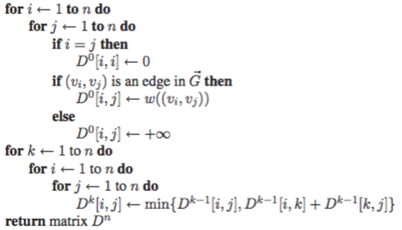Develop New Solver

Steps to create a new solver: Following content is based on numerous documents available online. Some of the references are: OpenFOAM Programming – the basic classes by Prof Gavin Tabor, Introductory OpenFOAM Course University of Genoa, DICCA Dipartimento di Ingegneria Civile, Chimica e Ambientale, The Open Source CFD Toolbox - Programmer’s Guide, Basic OpenFOAM Programming Tutorial: Adding Passive Scalar Transport Equation to icoFoam by Vuko Vukcevic on youTube, OpenFOAM programming tutorial by Tommaso Lucchini ...

###### Step-01
Copy the existing solver nearest matching your needs from \$FOAM_APP folder to your local folder \$WM_PROJECT_USER_DIR. E.g. if you want to add new capability in combustion, XiFoam could be the sover. Thus, copy the files/folder \$FOAM_APP/solvers/combustion/XiFoam to \$WM_PROJECT_USER_DIR/userXiFoam. If \$WM_PROJECT_USER_DIR is not created, manually create folders to match the default path setting. e.g. the path defined for \$WM_PROJECT_USER_DIR on my machine is /home/AK/OpenFOAM/AK-7 where AK is the user name and '7' refers to OpenFOAM version. \$WM_PROJECT_DIR/applications contains following sub-directories and files:
• solvers: folder for the source code for the distributed solvers.
• test: folder for the source code of several test cases that show the usage of few OpenFOAM classes.
• utilities: folder for the source code for the distributed utilities.
• Allwmake: script file which compiles all the content of solvers and utilities. To compile the test cases in 'test' folder, move to the identified 'test' case directory and compile it by typing 'wmake'.
• foamNewBC and foamNewApp utilities can be used to create a directory with source and compilation files for a new application. e.g. foamNewBC -f -v expInlet will create a folder 'expInlet' in the working directory (directory from which command was issued) and create expInletFvPatchVectorField.C, expInletFvPatchVectorField.H and make directory.

Alternatively, if you want to start from ground zero, use command 'foamNewApp mySolver'. It will create a folder mySolver having subfolder Make. It will also create a file called mySolver.C. Note that it does not create any header file like mySolver.H or createFields.H. The source file mySolver.C will have empty 'Description' field. However, this is the hardest way to develop a solver as almost all controls (e.g. PISO) needs to be typed in. This is apparent from the source code of pimpleFoam that has been added in previous paragraphs.

###### Step-02
Clean all the dependancies using utility 'wclean'. Rename the *.C and *H files to any other name not in conflict with OpenFOAM solvers. In this case, XiFoam.C and bEqn.H should be renamed, say to userXiFoam.C and userBEqn.H. Update the name of application and description in the header section of the source code userXiFoam.c. OpenFOAM follows programming standard to organize the class files in pairs, one with the class declarations (*.H files) and other with the class definitions (*.C files).
###### Step-03
Update the 'make' file with new source file name. E.g.
```Existing 'make' file         |  New 'make' file
XiFoam.C                     |  userXiFoam.C

EXE = \$(FOAM_APPBIN)/XiFoam  |  EXE = \$(FOAM_USER_APPBIN)/userXiFoam ```
This can be done manually or by the commands "sed -i s/"XiFoam"/"userXiFoam"/g Make/files", "sed -i s/"FOAM_APPBIN"/"FOAM_USER_APPBIN"/g Make/files" and "sed -i s/"bEqn.H"/"userBEqn.H"/g *.*in" the Linux terminal.

\$(FOAM_USER_APPBIN)/userXiFoam instructs the compiler to name the application userXiFoam and to copy the executable in the directory \$FOAM_USER_APPBIN.

###### Step-04
Run 'wmake' command to ensure that the new set-up is updated and working as exact replica of the existing solver.
###### Step-05
Write your header files userHeader.H to implement the additional equations to be incorporated into the main solver. The existing header file createFields.H can also be used. Define any new variables which need to be added. For example, the phi field caculated and defined in createFields.H is described below. An IOobject gets modified during the solution.
```Info<< "Reading field phi (passive scalar)\n" << endl;
surfaceScalarField phi (
IOobject (  //phi is an input/ouput object type
"phi",
runTime.timeName(),  //The variable is bound to runtime and mesh
mesh,
IOobject::AUTO_WRITE
),
fvc::flux(U)
); ```
runTime.timeName() implies that variable is bound to runtime and mesh. IOobject::NO_READ - it does not need to be read at the start of runtime. IOobject::MUST_READ should be used for variables which needs to be solved such as fields 'p' and 'U'. This enforces the boundry conditions to be imposed. IOobject::AUTO_WRITE - instructs the solver to write the variable when runtime equals the write frequency defined in controlDict. The variable viscosity is defined below which is a scalar, named as 'nu' and can be found in the dictionary 'transportProperties'. If a similar variable, say xeta is to be defined, copy this piece of code and modify.
```dimensionedScalar nu (
"nu",
dimViscosity,
transportProperties.lookup("nu")
); ```

###### Step-06
Add #include "userHead.H" in the main solver file userXiFoam.C after the statement runTime.write(). If you want to create a new boundry conditions, copy appropriate boundary definition from folder src/finiteVolume/fields/fvPatchFields/derived.
###### Step-07
Add appropriate headers and new scalars/vectors needed to implement the new equation
###### Step-08
Update dictionaries with new variables, create a file with the name of variable in '0' folder, update discretization schemes (divergence - divSchemes, Laplacian - laplacianSchemes for new variables) in 'fvSchemes' dictionary. Define the solver for new field variables in dictionary 'fvSolution'.
```divSchemes {
default          none;
div(phi,k)       bounded Gauss upwind;
...
```
```U {
solver          smoothSolver;
smoother        symGaussSeidel;
tolerance       1e-05;
relTol          0;
} ```

#### Implementation of a new user-defined B.C.Step-00:

Check that the folders \$WM_PROJECT_USER_DIR and \$FOAM_USER_LIBBIN are available using commands 'cd \$WM_PROJECT_USER_DIR' and 'cd \$FOAM_USER_LIBBIN'. If you get error: "No such file or directory", use 'echo' command to get the path defined for \$WM_PROJECT_USER_DIR and \$FOAM_USER_LIBBIN. Create the missing folder. E.g. in "Windows Subsystem for Linux" on my machine, \$FOAM_USER_LIBBIN is defined as \$WM_PROJECT_USER_DIR/ platforms/ linux64GccDPInt32Opt/ lib.

Step-01:

Create a local folder for user specific B.C. such as 'myBC' under \$WM_PROJECT_USER_DIR directory. Copy the nearest matching boundary condition file from the folder \$FOAM_SRC/ finiteVolume/ fields/ fvPatchFields/ derived to myBC. E.g. if you want to implement a scalar field B.C. copy scalar field based such as totalTemperature. Rename totalTemperatureFvPatchScalarField.C and totalTemperatureFvPatchScalarField.H to myNewBcFvPatchScalarField.C and myNewBcFvPatchScalarField.H respectively.

foamNewBC utility can be used to create a directory with source and compilation files for a new application. e.g. foamNewBC -f -v expInlet will create a folder 'expInlet' in the working directory (directory from which command was issued) along with additional files expInletFvPatchVectorField.C and expInletFvPatchVectorField.H as well as make directory. expInletFvPatchVectorField.C: is the actual source code of the application. This file contains the definition of the classes and functions. expInletFvPatchVectorField.H: header files required to compile the application. This file contains variables, functions and classes declarations.

Step-02:

Copy 'Make' from \$FOAM_SRC/finiteVolume to \$WM_PROJECT_USER_DIR/myBC folder. Remove all lines except the one containing 'totalTemperature' term. Alternatively, one can start with a cleaned version of 'files'. It will contain only following 2 lines:

\$(derivedFvPatchFields)/totalTemperature/totalTemperatureFvPatchScalarField.C

LIB = \$(FOAM_LIBBIN)/libfiniteVolume

Rename or replace the keyword 'totalTemperature' with avgControlled. \$(derivedFvPatchFields) needs to be deleted as the new *.C and *H files are located in local folder. In VIM editor, one can use the command ":%s/totalTemperature/avgControlled/g". Thus, the content of 'files' will change to:

avgControlled/avgControlledFvPatchScalarField.C

LIB = \$(FOAM_USER_LIBBIN)/libfiniteVolume

The content of 'options' file will change as follows:

Official release file

```
EXE_INC = \
-I\$(LIB_SRC)/triSurface/lnInclude \
-I\$(LIB_SRC)/meshTools/lnInclude  \

LIB_LIBS =     \
-lOpenFOAM   \
-ltriSurface \
-lmeshTools
```

Modified file

```EXE_INC = \
-I\$(LIB_SRC)/triSurface/lnInclude \
-I\$(LIB_SRC)/meshTools/lnInclude  \
-I\$(LIB_SRC)/finiteVolume/lnInclude

LIB_LIBS =     \
-lOpenFOAM   \
-ltriSurface \
-lmeshTools  \
-lfiniteVolume
```

Step-03:

Update the header file *.H and source file *.C by replacing all the occurrence of 'totalTemperature' with 'avgControlled'. The actual implementation of the boundary condition is done by the updateCoeffs() member function. In the file uniformInletOutlet.H, the TypeName("uniformInletOutlet") is used to define the boundary condition name.

Notice the following sections in the expInletFvPatchVectorField.H file. Different types of private data are declared. These are the variables required for the implementation of the new BC. In implementation of exponential velocity profile, we need to use vectors and scalars, therefore we can keep the lines scalar scalarData_; and vector data_; Other lines can be deleted as those datatypes are not needed.

Also, as we will use two vectors in our implementation, we need to duplicate lines containing "vector data_" (total 6 occurrences). Note that all data members should be of type 'private'. The OpenFOAM convention is to have trailing underscore in the names.

```// Private Data
//- Single valued scalar quantity, e.g. a coefficient
scalar scalarData_;
//- Single valued Type quantity, e.g. reference pressure pRefValue_
// Other options include vector, tensor
vector data_;

//- Field of Types, typically defined across patch faces
// e.g. total pressure p0_. Other options include vectorField
vectorField fieldData_;

//- Type specified as a function of time for time-varying BCs
autoPtr<Function1<vector>> timeVsData_;

//- Word entry, e.g. pName_ for name of the pressure field on database
word wordData_;

//- Label, e.g. patch index, current time index
label labelData_;

//- Boolean for true/false, e.g. specify if flow rate is volumetric_
bool boolData_; ```

Step-04:

expInletFvPatchVectorField.H: Change the name of scalarData_ to meanVelocity_ and change the names of the two vectors 'data_' to 'n_' and 'R_' respectively. It is recommended to initialize them in the same order as you declare them in the header file. As shown below, the vectors n and y are initialized as a Zero vector (shortcut for (0, 0, 0)) by default. It is not recommended to initialize these vectors as zero vectors by default. Initialize vector n_ and vector R_ as (1, 0, 0) and (0,1,0) respectively.

Original section of code

```    fixedValueFvPatchVectorField(p, iF),
meanVelocity_(0.0),
n_(Zero),
R_(Zero)```

Modified section of code

```    fixedValueFvPatchVectorField(p, iF),
meanVelocity_(0.0),
n_(1, 0, 0),
R_(0, 1, 0)```

Step-05:

expInletFvPatchVectorField.C: Notice the following function that allows access to simulation time. Since in this implementation of exponential velocity profile, we do not need to use time these lines can be deleted. Since the datatypes fieldData, timeVsData, wordData, labelData and boolData were deleted in the header file, delete them in the C file too. Erase only the lines that contain the words fieldData, timeVsData, wordData, labelData and boolData such as following lines:
```Foam::expInletFvPatchVectorField::
expInletFvPatchVectorField (
const fvPatch& p,
const DimensionedField<vector, volMesh>& iF
)
:
fixedValueFvPatchVectorField(p, iF),
scalarData_(0.0),
data_(Zero),
fieldData_(p.size(), Zero),
timeVsData_(),
wordData_("wordDefault"),
labelData_(-1),
boolData_(false) {
}```
Similarly, delete 5 more occurrences of similar lines - lines that contain the words fieldData, timeVsData, wordData, labelData and boolData. Since this implementation do not need to use time, remove the following lines as well.
```Foam::scalar Foam::expInletFvPatchVectorField::t() const {
return db().time().timeOutputValue();
}```
Also delete the line "writeEntry(os, timeVsData_())" under block 'void Foam::expInletFvPatchVectorField::write'.

Another option is to search for the words fieldData, wordData, labelData and boolData to delete all these lines. In editor 'vim', use :g/fieldData/d to delete all lines containing "profile" (remove /d i.e. use :g/fieldData/ to check or view the lines that will get deleted).

Step-06:

Replace all the occurrences of the word scalarData with meanVelocity (11 occurrences). In 'Vim', use the command ":%s/scalarData/meanVelocity/g". Duplicate all the lines (ignore if this task was already performed in step-3) where the word 'data' appears (6 lines), change the word 'data' to n in the first line and to R in the second line, erase the comma in the last line (6 occurrences).

dict.lookup will look for these keywords in the input dictionary

```fixedValueFvPatchVectorField(p, iF),
meanVelocity_(dict.lookup<scalar>("meanVelocity")),
n_(pTraits<vector>(dict.lookup("n"))),
R_(pTraits<vector>(dict.lookup("R"))) ```

Step-07:

Delete the entries in the block: fixedValueFvPatchVectorField::operator== that is delete the following lines. The access function operator == is used to assign the values to the boundary patches. This section shall be replaced with new boundary condition expressions.
```(
data_
+ fieldData_
+ scalarData_*timeVsData_->value(t())
); ```

Step-08:

Implement the actual boundary condition in void Foam::fixedValueFvPatchVectorField::updateCoeffs(). Add the following lines after if (updated()) { return; }. The implementation of the boundary condition is always done using the updateCoeffs() member function.
```//Get dimensions of the bounding box: MIN and MAX points
boundBox bb(patch().patch().localPoints(), true);

//Calculate coordinates of centre point of the patch
vector ctr = 0.5*(bb.max() + bb.min());
vector Dh = (bb.max() - bb.min());

//Access centre of the faces in the patch
const vectorField& c = patch().Cf();

//Computes scalar field to be used for defining the parabolic profile
//& denotes dot product of two vectors in OpenFOAM
scalarField coord = 2*((c - ctr) & R_)/(Dh & R_); ```

Step-09:

Add the following statement under block fixedValueFvPatchVectorField::operator== which was deleted in step-07. The access function operator == is used to assign the values to the boundary patches.

n_*meanVelocity_*(1.0 - sqr(coord))

Step-10:

Use 'wmake' to compile (and check) new source codes. It will compile a library with the name specified in the file Make/file. In this case, the name of the library is libexpInlet (expInlet was the name of B.C. chosen). The library will be located in the directory \$FOAM_USER_LIBBIN as specified in the file Make/file.

Step-11:

If everything is working fine thus far, add sanity checks on new variables n_ and R_ as you deem appropriate. For example, the value of n and R should be > 0. Before block fixedValueFvPatchVectorField::evaluate() add the following line of codes.
```if (mag(n_) < SMALL || mag(R_) < SMALL) {
FatalErrorIn("expInletFvPatchVectorField(dict)")
<< "n and/or R given with zero  or close to zero."
<< abort(FatalError);   //Abort exeuction - solver will exit
}
n_ /= mag(n_); //This is equivalent to n_ = n_/mag(n_)
R_ /= mag(R_); //This is equivalent to R_ = R_/(mag(y_)```

Step-12:

Re-complile and the boundary condition is ready to be used. Go to the case directory, open file 0/U and update the definition of the new B.C. 'vIn'. We also need to tell the application that we want to use a new library. To add the new library in the dictionary file controlDict need to be updated.
```vIn  {
type         expInlet;  //Name of the boundary condition
meanVelcoity 2.5;       //User defined values
n            (1 0 0);
y            (0 1 0);
} ```

Add the name of the library in the controlDict.

libs ("libexpInlet.so");

Step-13:

You may add verbosity (extra information for user). This can be done by adding statements after the function updateCoeffs. Any new changes to expInletFvPatchVectorField.C shall need to be re-compiled.

Step-14:Here is the final version of source file expInletFvPatchVectorField.C

These steps are based on user instruction document from WolfDynamics: "Introductory OpenFOAM Course From 16th to 20th July, 2018", University of Genoa, Dipartimento di Ingegneria Civile, Chimica e Ambientale. Different names have been used to avoid following the code verbatim as mentioned in this document. Using different variable names necessitated or enforced user to carefully look into the codes.

#### Porous Media

The porous media source files are located in the directory: \$FOAM_SRC/ finiteVolume/ cfdTools/ general/ porousMedia/ and contains the following files: porousZones.H, porousZones.C, porousZone.H, porousZone.C, porousZonesTemplates.C - implementation of the porosity equations, porousZoneTemplates.C

The viscous and inertial source terms are defined in the member function addViscousInertialResistance and the power law from is defined in the addPowerLawResistance member function.

#### Cavitation Models

Reference: Implementing a Zwart-Gerber-Belamri cavitation model, Marcus Jansson, Applied Thermal and Fluid Sciences, Linkoping University

The dynamics of a single bubble subjected to a pressure field can be described by following which is known as the Rayleigh-Plesset equation. R = R(t) is the bubble radius, S is the surface tension coeffcient, pb is the bubble pressure and p∞ is the far-field pressure. ρl and νl is the liquid density and liquid kinematic viscosity respectively. The Rayleigh-Plesset equation assumes that bubbles are spherical and symmetric and that thermal effects are negligible. The equations of a cavitation model are:Note that Pv is designated as pSat in C++ code. Subscript 'nuc' stands for nucleation. Mass transfer is decomposed to vaporization- and condensation terms where mα- and mα+ are the destruction of liquid from evaporation and creation of liquid from condensation, respectively.αl is the liquid volume fraction. ZGB model assumes a constant nucleation site volume. Thus, both the nucleation site volume fraction rnuc and nucleation site radius RB are model constants. According to Zwart et. al. the default values of these constants are rnuc = 5.0E-4 and RB = 1.0E-6 [m] respectively.
• Solves for two isothermal, incompressible fluids using a Volume of Fluids (VoF) approach where momentum equations are only solved for the mixture.
• Mass transfer through cavitation with the models Merkle, Kunz and Schnerr-Sauer.
• Solves for the liquid fractiona and includes effect of gravity.
• interPhaseChangeFoam uses alphaEqnSubCycle to correct the phase interfaces.
• alphaEqnSubCycle (and alphaEqn) includes vDotAlphal which is part of the phaseChangeTwoPhaseMixtures library.
• vDotAlphal includes mDotAlphal which is different for every cavitation model
• Member functions: rRb, alphaNuc, pCoeff, mDotAlphal, mDotP - as described in the Rayleigh-Plesset and Schnerr-Sauer model equations above.
• The volume source terms vDotvAlphal (vaporization) and vDotcAlphal (condensation) are components of the pair called vDotAlphal. This is found in the phaseChangeTwoPhaseMixtures class, which is a part of the interPhaseChageFoam solver.
Step-1: Copy the existing cavitation models in phaseChangeTwoPhaseMixtures to your user directory. Copy the Schnerr-Sauer cavitation model and rename the files.
• cp -r \$FOAM_APP/ ... /phaseChangeTwoPhaseMixtures \$WM_PROJECT_USER_DIR/ ... /phaseChangeTwoPhaseMixtures
• cd \$WM_PROJECT_USER_DIR/src/phaseChangeTwoPhaseMixtures
• cp -r SchnerrSauer Zwart
• Rename Schnerr-Sauer files: cd Zwart, mv SchnerrSauer.C Zwart.C, mv SchnerrSauer.H Zwart.H

Step-2: Replace each 'SchnerrSauer' with 'Zwart' in Zwart.C and Zwart.H. Use Linux command: sed -i s/"SchnerrSauer"/"Zwart"/g Zwart.*

Step-3: Update wmake 'files' such that it ends with the following two lines:

```...
Zwart/Zwart.C
LIB = \$(FOAM_USER_LIBBIN)/libphaseChangeTwoPhaseMixtures```

Step-4: Zwart.H - Remove the private member data for n_, dNuc_, rRb_ and alphaNuc in Zwart.H Add the new member data between Cv_ and p0_.

```//- Nucleation site volume
dimensionedScalar rNuc_;
dimensionedScalar Rb_; ```

Step-5: Zwart.C - Remove the constructors for n_ and dNuc_. Add in the constructor, between Cv_ and p0_: rNuc_("rNuc", dimless, phaseChangeTwoPhaseMixtureCoeffs_), Rb_("Rb", dimLength, phaseChangeTwoPhaseMixtureCoeffs_),

Step-6: Zwart.C - Remove the entire member functions rRb and alphaNuc. Replace the member functions pCoeff and mDotAlpha

pCoeff: Zwart Model

```Foam::tmp<Foam::volScalarField>
Foam::phaseChangeTwoPhaseMixtures::Zwart::pCoeff (
const volScalarField& p
)
const {
return (3*rho2())*sqrt(2/(3*rho1())) /(Rb_*sqrt(mag(p - pSat()) + 0.01*pSat()));
} ```

mDotAlphal (Schnerr-Sauer Model)

```Foam::Pair<Foam::tmp<Foam::volScalarField>>
Foam::phaseChangeTwoPhaseMixtures::SchnerrSauer::mDotAlphal() const  {
const volScalarField& p = alpha1_.db().lookupObject<volScalarField>("p");
volScalarField limitedAlpha1(min(max(alpha1_, scalar(0)), scalar(1)));
volScalarField pCoeff(this->pCoeff(p));
return Pair<tmp<volScalarField>>
(
Cc_*limitedAlpha1*pCoeff*max(p - pSat(), p0_),
Cv_*(1.0 + alphaNuc() - limitedAlpha1)*pCoeff*min(p - pSat(), p0_)
);
} ```

mDotAlphal (Zwart Model)

```Foam::Pair<Foam::tmp<Foam::volScalarField>>
Foam::phaseChangeTwoPhaseMixtures::Zwart::mDotAlphal() const {
const volScalarField& p = alpha1_.db().lookupObject<volScalarField>("p");
volScalarField limitedAlpha1(min(max(alpha1_, scalar(0)), scalar(1)));
volScalarField pCoeff(this->pCoeff(p));
return Pair<tmp<volScalarField>>
(
Cc_*pCoeff*max(p - pSat(), p0_),
Cv_*rNuc_*pCoeff*min(p - pSat(), p0_)
);
} ```

mDotP (Zwart Model)

```Foam::Pair<Foam::tmp<Foam::volScalarField>>
Foam::phaseChangeTwoPhaseMixtures::Zwart::mDotP() const {
const volScalarField& p = alpha1_.db().lookupObject<volScalarField>("p");
volScalarField pCoeff(this->pCoeff(p));
volScalarField limitedAlpha1(min(max(alpha1_, scalar(0)), scalar(1)));
return Pair<tmp<volScalarField>>
(
Cc_*(1.0 - limitedAlpha1)*pos(p - pSat())*pCoeff,
(-Cv_)*rNuc_*limitedAlpha1*neg(p - pSat())*pCoeff
);
} ```

Step-7: remove n_ and dNuc_ from the read function and add rNuc_ and Rb_.

```bool Foam::phaseChangeTwoPhaseMixtures::Zwart::read() {
phaseChangeTwoPhaseMixtureCoeffs_ = optionalSubDict(type() + "Coeffs");
phaseChangeTwoPhaseMixtureCoeffs_.lookup("Cc") >> Cc_;
phaseChangeTwoPhaseMixtureCoeffs_.lookup("Cv") >> Cv_;
phaseChangeTwoPhaseMixtureCoeffs_.lookup("rNuc") >> rNuc_;
phaseChangeTwoPhaseMixtureCoeffs_.lookup("Rb") >> Rb_;
return true;
} else {
return false;
}
} ```

Step-8: Compile the class again using wmake. Go to the run directory and copy the throttleSchnerr tutorial. Remove the old time directories. In constant/ transportProperties, change phaseChangeTwoPhaseMixture from SchnerrSauer to Zwart to use the new cavitation model. Add the new model coeffcients after the section with SchnerrSauerCoeffs. Note the addition of rNuc and rRb and the values for Cc and Cv.

```ZwartCoeffs {
Cc 	0.01;
Cv 	50;
rNuc 	5.0e-04;
Rb 	1.0e-06;
} ```

Step-9: Solve the case, now with Zwart-Gerber-Belamri cavitation model by typing 'interPhaseChangeFoam' on command prompt.

#### Implement Non-Newtonian Viscosity Model

The Casson model is a non-Newtonian viscosity model used for chocolate, blood... The step-by-step procedure to implement it in OpenFOAM is described below.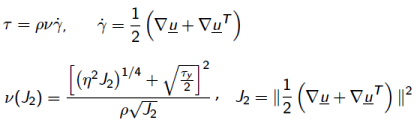Reference: How to write OpenFOAM Apps and Get on in CFD, 10th OpenFOAM Workshop, U. Michigan, Ann Arbor, Dr Gavin Tabor, 30-June-2015.

Step-01:

Seach the nearest matching model, e.g. Power Law in this case. Copy powerLaw sub-directory to user directory and rename the files Casson.H and Casson.C.

Step-02:

Change all instances of powerLaw to Casson inside the *.C and *.H files. Change private data to hold the Casson model coefficients. Update constructor, nu() and correct() functions.

Step-03:

Convert the viscosity 'nu' from dimensionedScalar into volScalarField and remove the original definition of 'nu' as a dimensionedScalar.

Info << " Reading field nu" << nl << endl;

```volScalarField nu (
IOobject (
"nu", runTime.timeName (),
mesh,
), mesh
);```

Step-04:

Calculate the value of nu somewhere within the iterative loop to read in Casson model cofficients in createFields.H. Here keyword 'tiny' is used to avoid division by zero.

volScalarField J2 = 0.5* magSqr(symm (fvc::grad (U ))) + dimensionedScalar ("tiny", dimensionSet (0,0,-2,0,0,0,0), 0.0001);

nu = sqr(pow(sqr(eta)*J2, 0.25) + pow(tau_y/2, 0.5)) / (rho*sqrt(J2));

Step-05:

Update 'files' and 'options' in Make directory. Compile using wmake.

Step-06:

Select new viscosity model by adding the line libs("libCassonModel.so"); in controlDict.

Step-07:

Specify the coefficients in the dictionary 'transportProperties'.
```transportModel Casson;
CassonCoeffs {
eta   eta   [1 -1 -1 0 0 0 0 ]  5.00;
tau_y tau_y [1 -1 -2 0 0 0 0 ]  12.0;
rho   rho   [1 -3  0 0 0 0 0]   1100;
}```

Step-08:

Run a case with nonNewtonianIcoFoam.

#### Dynamic MeshA good report on Implementation of dynamicMesh in OpenFOAM can be found here. The implementation of point-wise deformation of meshes can be found in this tutorial document.

Example implementation in rhoPimpleFoam and explanations are described below.

```#include "fvCFD.H"
#include "dynamicFvMesh.H"  //Inclusion of classes to handle the dynamic mesh
#include "psiThermo.H"
#include "turbulenceModel.H"
#include "bound.H"
#include "pimpleControl.H"
#include "fvIOoptionList.H"
// * * * * * * * * * * * * * * * * * * * * * * * * * * * //
int main(int argc, char *argv[])
{
#include "setRootCase.H"
#include "createTime.H"

//Creates mesh ojbect as a dynamicFvMesh and not as fvMesh
#include "createDynamicFvMesh.H"
#include "initContinuityErrs.H"

pimpleControl pimple(mesh);

#include "createFields.H"
#include "createFvOptions.H"
#include "createPcorrTypes.H"
#include "createRhoUf.H"
Includes:
surfaceVectorField rhoUf (
IOobject (
"rhoUf",
runTime.timeName(),
mesh,
IOobject::AUTO_WRITE
),
fvc::interpolate(rho*U)
);

#include "CourantNo.H"
#include "setInitialDeltaT.H"

//-----------------------------------------------------------------------------
while (runTime.run()) {
{
//Absolute convective flux due to the flow motion
volScalarField divrhoU (
"divrhoU",
fvc::div(fvc::absolute(phi, rho, U))
);
runTime++;
volVectorField rhoU("rhoU", rho*U);

// Do any mesh changes: Triggers the mesh motion updating the new point
// positions and calculating the mesh flux
mesh.update();

if (mesh.changing() && correctPhi) {
phi = mesh.Sf() & rhoUf;
#include "correctPhi.H"
// Make the fluxes relative to the mesh-motion
fvc::makeRelative(phi, rho, U);
}
}
} ```

#### Electromagnetics

Variables in electromagnetism:

• Q: Electric charge [C]
• A: magnetic potential vector
• H: magnetic field strength (A/m or N/Wb or Oersted)
• J: current density [A/m2]
• D: electric flux density or electric displacement [C/m2]
• E: electric field strength or electric field intensity [V/m]
• B: magnetic flux density [T] or [Wb/m2]
• ε: absolute electrical permittivity, ε0 = absolute permittivity of vacuum = 8.854E-12 [F/m]
• εr: relative electrical permittivity or Dielectric Constant (defined as 'k' in electrostaticFoam)
• μ: absolute magentic permeability (subscript '0' indicates permeability of vacuum or air = 4π×10-7 H/m)
• ρ: volume density of charge (∇D = ρ) or electrical resistivity (electrical resistance, R = ρ × Length / Area) - [Ω-m] or [Ohm-m]
• σ: electrical conductivity = 1/ρ - [1/Ω-m]
Boundary conditions at interfaces: there are discontinuities in electric fields at the boundaries between conductors and dielectrics due to different permittivities. The relationship defined between electric field strenths and flux densities at such boundaries are called the boundary conditions. The first boundary condition is that the normal component of flux density is continuous across a surface: D1N = D2N. The second boundary condition is that the tangential field strength is continuous across the boundary : E = E.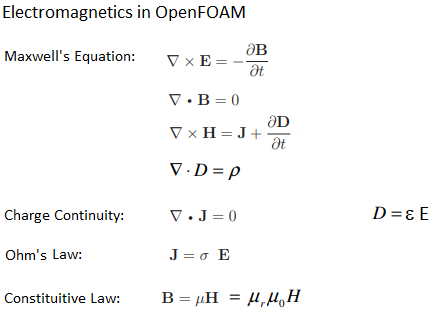Combining above equations and assuming Coulomb gauge condition (∇.A = 0) results in Poisson equation for the magnetic potential and a Laplace equation for the electric potential (E, ElPot). Note that field variable φ is denoted by ElPot in OpenFOAM code.

Magnetic Potential Equation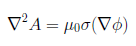```B = fvc::curl(A);
solve (
fvm::laplacian(A) == muMag * sigma * (fvc::grad(ElPot))
); ```

Electric Potential Equation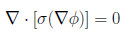```solve (
fvm::laplacian(sigma, ElPot)
); ```

As on Feb 2021 and OpenFOAM V8, the standard release contains 3 solvers dealing with magnetic field.
1. magneticFoam: solver for the magnetic field generated by permanent magnets
2. electrostaticFoam: solver for electrostatics - the static current (current does not change with time). Note that current is flow of charge. The equations which are solved are:```solve (
fvm::laplacian(phi) + rho/epsilon0
); ```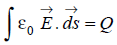```rhoFlux = -k*mesh.magSf()*fvc::snGrad(phi);
solve (
fvm::ddt(rho) + fvm::div(rhoFlux, rho)
);```
3. mhdFoam: Solver for magnetohydrodynamics (MHD) - incompressible, laminar flow of a conducting fluid under the influence of a magnetic field. An applied magnetic field H acts as a driving force, at present boundary conditions cannot be set via the electric field E or current density J. The fluid viscosity nu, conductivity sigma and permeability mu are read in as uniform constants.

A fictitious magnetic flux pressure pB is introduced in order to compensate for discretisation errors and create a magnetic face flux field which is divergence free as required by Maxwell's equations.

However, in this formulation discretisation error prevents the normal stresses in UB from cancelling with those from BU, but it is unknown whether this is a serious error. A correction could be introduced whereby the normal stresses in the discretised BU term are replaced by those from the UB term, but this would violate the boundedness constraint presently observed in the present numerics which guarantees div(U) and div(H) are zero.

#### ELECTROMAGNETIC FOAM

Step by step approach to implement a solver for electromagnetic calculation is described below.

Step-01: Create a folder say 'eMagFoam' in your \$WM_PROJECT_USER_DIR directory: cd \$WM_PROJECT_USER_DIR ❯ mkdir eMagFoam ❯ cd eMagFoam. Note that foamNewSource shall create app (solver) name based on the folder

Step-02: Generate a new solver template files using command - foamNewSource App emagFoam. This will create emagFoam.C file and Make folder. The Make/files will contain following two lines:

```eMagFoam.C

EXE = \$(FOAM_APPBIN)/eMagFam```
Change last line to: EXE = \$(FOAM_USER_APPBIN)/eMagFoam. The 'Make/options' file will contain following two lines:
```EXE_INC = \
-I\$(LIB_SRC)/finiteVolume/lnInclude

EXE_LIBS = \
-lfiniteVolume```
Include the two libraries: -I\$(LIB_SRC)/meshTools/lnInclude and -lmeshTools. Thus, the Make/options will contain now:
```EXE_INC = \
-I\$(LIB_SRC)/finiteVolume/lnInclude \
-I\$(LIB_SRC)/meshTools/lnInclude

EXE_LIBS = \
-lfiniteVolume \
-lmeshTools```

Step-03: Update the eMagFoam.C file by adding following two include statments. Note that the createMesh.H should be included before 'createField.H' else compilation step (wmake) will throw error.

#include "createMesh.H"

#include "createFields.H"

Add the solve statements to solve PDEanddescribed earlier.

```solve (
fvm::laplacian(sigma, ElPot)
);

solve (
fvm::laplacian(A) == muMag * sigma * (fvc::grad(ElPot))
);```
One can calculate additional variables such as magnetic flux density: B = fvc::curl(A);

Step-04: Add statements to write the results into a new 'time' directory.

runTime++; sigma.write(); ElPot.write(); A.write(); B.write();

Step-05: construct and initialize muMag, sigma, Elpot, A, B in createFields.H which will contain the statements and expression as per this file.

Step-06: Compile the new solver using 'wmake'. If the process is successful, you will get following statement as last line of the output: -lm -o /home/X/ OpenFOAM/X-8/ platforms/linux64GccDPInt32Opt/ bin/eMagFoam where 'X' stands for the user name.

Step-07: Create mesh, A, ElPot and sigma dictionaries in 0/ folder. Update the controlDict with solver eMagFoam. Run the case. Note the units of A (Magnetic Potential) is [1 1 -2 0 0 -1 0], ElPot (Electric Potential, φ) has unit of [1 2 -3 0 0 -1 0] and unit of sigma (electrical conductivity) is [-1 -3 3 0 2 0 0].

The magnetic field around a long straight conductor is explained below.Step-08: Check the result in ParaView.Use postProcess functionality to extract components such as (a) postProcess -func 'components(A)' -time 1 and (b)postProcess -func 'components(B)' -time 1.

The case file can be downloaded from here. Use the utilities blockMesh, changeDictionary, setFields and then solver eMagFoam in sequence.

Acoustics: Develop acoustic / noise models.

```acoustic = density0 * fvc::div(fvc::div(U*U));  //quadrupole distribution
monoPole = -fvc::div(psurfaceSource);
acousticSources =  monoPole + acoustic;
fvScalarMatrixpaEqn (
1.0/c/c * fvm::d2dt2(pa) - fvm::laplacian(pa); pa = p - pRef
);
solve(paEqn == acoustic);  // sound pressure as acoustic sources ```

#### Disclaimer:

This content is not approved or endorsed by OpenCFD Limited, the producer of the OpenFOAM software and owner of the OpenFOAM and OpenCFD trademarks.
##### References
1. OpenFOAM's basic solvers for linear systems of equations Solvers, preconditioners, smoothers by Tim Behrens, February 18, 2009
2. OpenFOAM Programmers Guide
3. Mathematics, Numerics, Derivations and OpenFOAM by Tobias Holzmann at Holzmann CFD
4. Dictionaries in tutorial folders of OpenFOAM